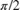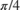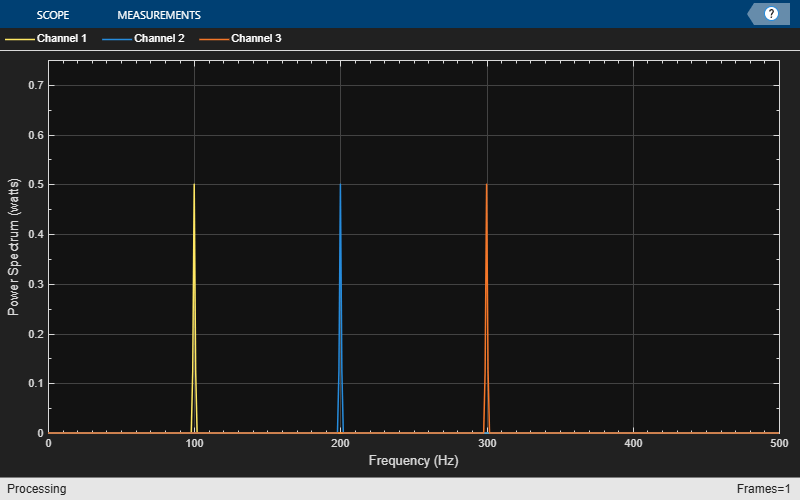getFrequencyVector

Vector of frequencies at which estimation is done

Description

example

freq = getFrequencyVector(estimator) returns the vector of frequencies at which the estimation is done.

freq = getFrequencyVector(estimator,Fs) returns the frequency vector assuming an input sample rate, Fs.

Examples

collapse all

Compute the power spectrum of a multichannel sinusoidal signal using the dsp.SpectrumEstimator System object™. You can get the vector of frequencies at which the spectrum is estimated using the getFrequencyVector function. To compute the resolution bandwidth of the estimate (RBW), use the getRBW function.

Generate a three-channel sinusoid sampled at 1 kHz. Specify sinusoidal frequencies of 100, 200, and 300 Hz. The second and third channels have their phases offset from the first byand, respectively.

sineSignal = dsp.SineWave('SamplesPerFrame',1000,'SampleRate',1000, ...
'Frequency',[100 200 300],'PhaseOffset',[0 pi/2 pi/4]);

Estimate and plot the one-sided spectrum of the signal. Use the dsp.SpectrumEstimator object for the computation and the dsp.ArrayPlot for the plotting.

estimator = dsp.SpectrumEstimator('FrequencyRange','onesided');
plotter = dsp.ArrayPlot('PlotType','Line','YLimits',[0 0.75], ...
'YLabel','Power Spectrum (watts)','XLabel','Frequency (Hz)');

Step through to obtain the data streams and display the spectra of the three channels.

y = sineSignal();
pxx = estimator(y);
plotter(pxx)Get the vector of frequencies at which the spectrum is estimated in Hz, using the getFrequencyVector function.

f = getFrequencyVector(estimator);

Compute the resolution bandwidth (RBW) of the estimate using the getRBW function.

rbw = getRBW(estimator)
rbw =

0.0015

The resolution bandwidth of the signal power spectrum is 0.0015 Hz. This frequency is the smallest frequency that can be resolved on the spectrum.

Input Arguments

collapse all

Estimator object, specified as one of the following:

Input sample rate, specified as a real positive scalar.

Output Arguments

collapse all

Spectrum frequencies, returned as a column vector.

The length of the frequency vector is determined by the FrequencyRange and the FFT length.

If you set the FrequencyRange to 'onesided' and the FFT length, NFFT, is even, the frequency vector is of length NFFT/2+1, and covers the interval [0,SampleRate/2].

If you set the FrequencyRange to 'onesided' and NFFT is odd, the frequency vector is of length (NFFT+1)/2 and covers the interval [0,SampleRate/2].

If you set the FrequencyRange to 'twosided', the frequency vector is of length NFFT and covers the interval [0, SampleRate].

If you set the FrequencyRange to 'centered', the frequency vector is of length NFFT and covers the range [-SampleRate/2, SampleRate/2] and [-SampleRate/2, SampleRate/2] for even and odd length NFFT, respectively.

Data Types: single | double

Introduced in R2013b

DSP System Toolbox DocumentationGet trial now